Maths-
General
Easy

Question

# How can you use polynomial identities to multiply expressions ?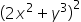Hint:

## The correct answer is: 4x4 + 4x2y2 + y6

### Step 1 of 2:Here, the given expression is, where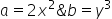.So, we can use the identity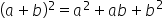to find the expansion ofStep 2 of 2:Apply the polynomial identity,to get the answer of.Thus, we have: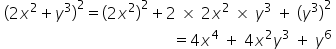Thus, the expansion is: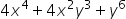Any expression of the form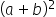can be expanded using the identityrather than using the conventional and time taking method of multiplying them,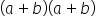.#### With Turito Foundation.#### Get an Expert Advice From Turito.